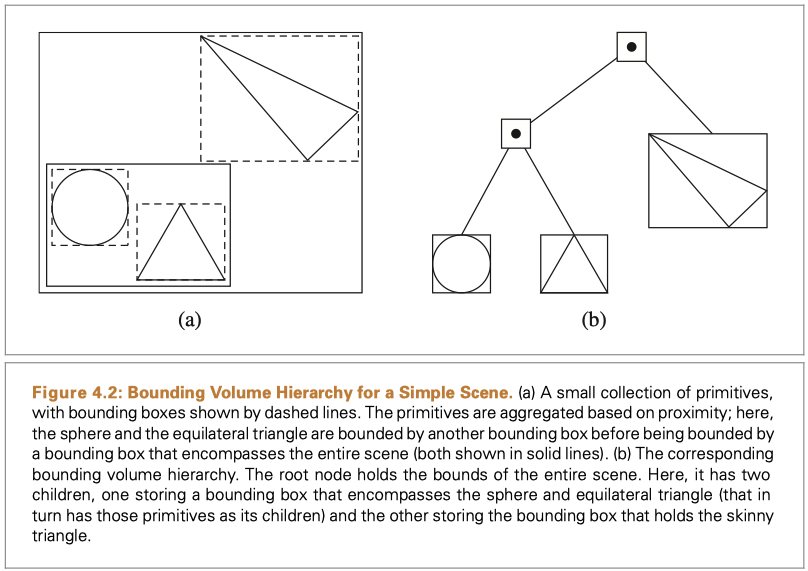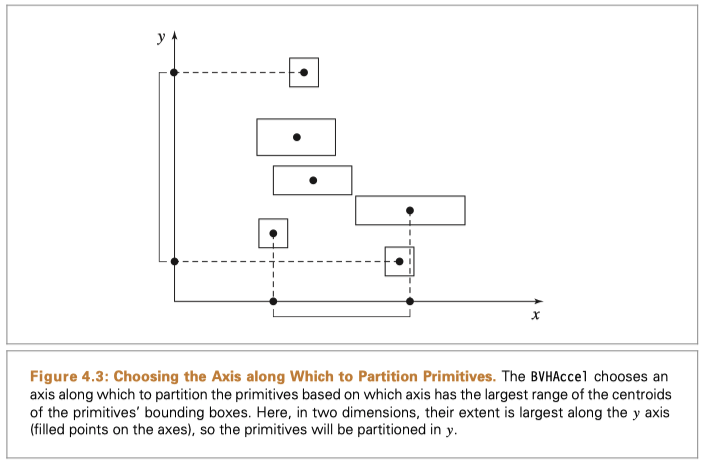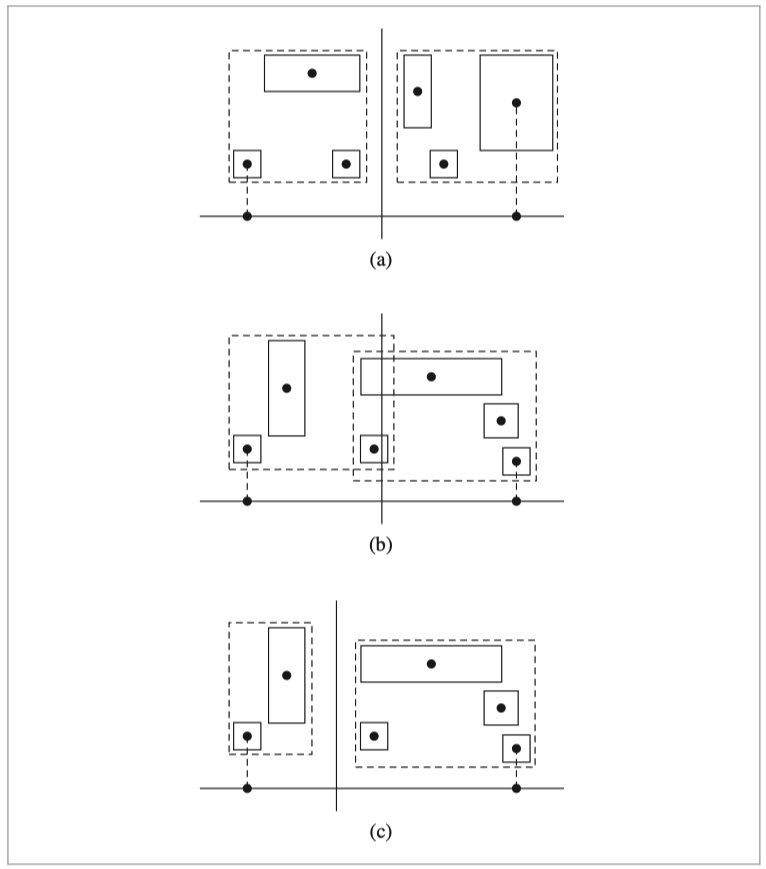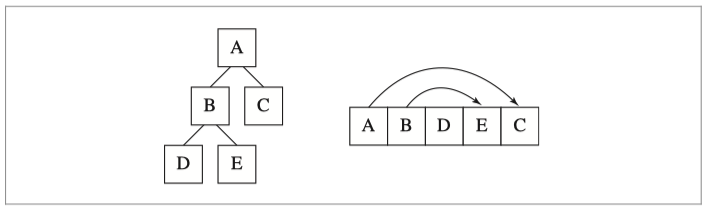0%

"Bounding volume hierarchies (BVHs) are an approach for ray intersection acceleration based on primitive subdivision, where the primitives are partitioned into a hierarchy of disjoint sets."

-- PBRT-v3

Intersection testing is an important part of the rendering, and thus the acceleration is concerned. Bounding volume hierarchies (BVHs) is a widely used method, which organizes the primitives of a scene with a binary tree. The core concept is that each node of the binary tree stores a bounding box that includes all primitives of the subtree. And therefore, the rendering engine can check the intersection quickly by traveling the tree and check the bounding boxes along the path. To check whether a ray hit a primitive, the engine uses a binary search to find the primitive while testing the intersection with the bounding boxes along the path. If one of the tests fails, then the ray must not hit the primitive we're looking for because the children nodes' volume must be the sub-volume of the parent node.Illustration of the BVHs

(Note: The copyright of some quotes, source code and images in this post belong to PBRT-v3.)

In comparison to spatially subdivision methods like KD-tree, we can estimate the maximum memory cost of BVHs. Because the height of the binary tree is related to the sum of the primitives. As binary BVH stores the primitive in the leaf (as you can see in the illustration above), for an n-primitives scene, the number of nodes is 2n-1. It is composed of n leaves and n-1 inner nodes that hold the bounding box information. In some implementations, a leaf stores more than 1 primitive, and the total nodes number is even smaller in such a situation. On the other hand, BVHs are generally more numerically robust to missed intersections in comparison to spatially division algorithms.

# Construction

There are three stages to construct a BVH in the implementation of PBRT-v3:

1. Computing bounding information about each primitive and storing them in an array to be used later.
2. Split the scene. Different algorithms could be applied here, and the choice will result in a different binary tree.
3. Convert the tree to a more compact pointerless representation for use during rendering to improve the efficiency.

For those who just want to practice BVHs instead of using it in rendering, step 3 will be optional.

The BVHs construction is implemented in

## Initialize primitive info array

Besides bounding information, some additional information is precomputed and stored in the array to reduce the computing cost in traveling. The declaration above is a local struct in accelerators/bvh.cpp. The meaning of member variables is apparent like the centroid is the "midpoint" of the bounding box.

The initialization code is straight-forward:

The WorldBound() method finally calls the one defined in shape and implemented in corresponding source files. Generally, it computes the bounding box first object coordinate and then applies a transform on it.

## Build BVH tree

With the primitive information array, now we can build the BVH tree via different algorithms. PBRT-v3 implements 4 algorithms, which are defined as:

If the user choose SplitMethod::HLBVH, the engine will call BVHAccel::HLBVHBuild while calling BVHAccel::recursiveBuild for the others. I will introduce the differences between them later. Now let's take a look at the general building pipeline first:

The struct BVHBuildNode is a util class and it is declared as:

It is similar to those node we often define in a binary tree. The only thing to notice I think is the difference operation applied to leaf and interior nodes. And we can see that all nodes of the BVH store a bounds variable to represent the bounding information.

### Recursive Build

The BVHAccel::recursiveBuild will construct a BVH with the primitives of the vector with index within [start, end). The main idea is:

1. Allocate the memory. (Use MemoryArena here, or you can just simply use new operator)

2. Compute the bounds of all primitives in the BVH node.

It is quite easy because you only need to union the bounds of all primitives belong to this node.

3. Determine how many primitives are contained in this node. If there's only one, create a leaf node, or create an interior node for other conditions.

(For creating a leaf node)

Get the corresponding index of the primitive from the primitveInfo vector, and then store the reference to the primitive to orderdPrims.

4. While creating an interior node, use a specific algorithm to choose the split dimension that divides the sequence [start ,end) to [start, mid) , [mid, end). After dividing, recursively call this function to build the children.

The main difference comes from the choice of dividing algorithm, there are $$2^n - 2$$ ways to partition n primitives into two non-empty groups. In practice when building BVHs, one generally considers partitions along a coordinate axis, meaning that there are about 6n candidate partitions. The implementation in PBRT-v3 chooses the axis associated with the largest extent when projecting the bounding box centroid for the current set of primitives.

The general goal in partitioning here is to select a partition of primitives that doesn’t have too much overlap of the bounding boxes of the two resulting primitive sets—if there is substantial overlap then it will more frequently be necessary to traverse both children subtrees when traversing the treeThe illustration explains what largest extent means.

A special case is that all centroid points overlap and thus the centroid bounds have zero volume. In such a case, there is no effective splitting method and therefore just put all the primitives in one single leaf node.

Now let's move on the different splitting methods:

1. SplitMethod::Middle: find a midpoint of the bounds centroid and then use it to divide the primitives into two sets by comparing the centroid with the midpoint. If the primitives all have large overlapping bounding boxes, this splitting method may fail to separate the primitives into two groups. In that case, execution falls through to the SplitMethod::EqualCounts approach to trying again.

The preference of the strategies is why to use switch instead of if else here, we expect the fall through to happen.

2. SplitMethod::EqualCounts: just easily divide the primitive to two $$n/2$$ subsets with std::nth_element(). But it fails in the situation (b) described below:Splitting Primitives Based on the Midpoint of Centroids on an Axis.

1. For some distributions of primitives, such as the one shown here, splitting based on the midpoint of the centroids along the chosen axis works well. (The bounding boxes of the two resulting primitive groups are shown with dashed lines.) (b) For distributions like this one, the midpoint is a suboptimal choice; the two resulting bounding boxes overlap substantially. (c) If the same group of primitives from (b) is instead split along the line shown here, the resulting bounding boxes are smaller and don’t overlap at all, leading to better performance when rendering.
3. SplitMethod::SAH: Surface Area Heuristic. It is the default splitting method because it provides a well-grounded cost model. The SAH model estimates the computational expense of performing ray intersection tests, including the time spent traversing nodes of the tree and the time spent on ray-primitive intersection tests for a particular partitioning of primitives. The main idea is dividing the bounds of the parent node to n (n = 8 here) buckets, and try a different ratio of buckets in two children then calculating the cost. Use the option with the lowest cost as the split point.

The corresponding source code is here. There is a comment at the beginning of each step, so it should be easy to understand now.

### Hierarchical Linear Bounding Volume Hierarchy (HLBVH)

Though SAH gives very good results, it is hard to parallelize, and many passes are taken over the primitives to compute the SAH costs. To address the problem, linear bounding volume hierarchies (LBVHs) were developed. It is much complicated than the methods I mentioned above, and it occupies many pages in the book. So that I plan to separate it to another post in the future to avoid putting too much content in this post. I think the construction methods mentioned above are enough for you to write your BVH, and you can read HLBVH if you want to improve it later.

## Compact BVH for Traversal

Now we have a BVH binary tree. Though you can just use the pointer to visit the tree, I'm gonna introduce another way to make the BVH pointerless, which increases the system performance with better use of cache and memory.Linear Layout of a BVH in Memory

The nodes of the BVH (left) are stored in memory in depth-ﬁrst order (right). Therefore, for any interior node of the tree (A and B in this example), the ﬁrst child is found immediately after the parent node in memory. The second child is found via an offset pointer, represented here with lines with arrows. Leaf nodes of the tree (D, E, and C) have no children.

(Illustration from PBRT-v3)

The structure LinearBVHNode is introduced to flatten the tree.

This structure is padded to ensure that it’s 32 bytes large. Doing so ensures that, if the nodes are allocated such that the ﬁrst node is cache-line aligned, then none of the subsequent nodes will straddle cache lines (as long as the cache line size is at least 32 bytes, which is the case on modern CPU architectures).

With the declaration of LinearBVHNode, we can allocate the memory needed at once.

Now let's move on to flattenBVHTree. The function is quite straightforward that the *offset parameter tracks the current offset of the array. The flatten function processes recursively:

1. Use the offset to get corresponding LinearBVHNode in the array, use (*offset)++ to assure the offset is unique.
2. For a leaf node, record the node->firstPrimOffset and node->nPrimitives.
3. For an interior node, flatten its two children recursively, and record the offset returned by its second child. (As the first child must be immediately after the current node because of the operation (*offset)++) In addition to bounds information, you need to record which axis to split as well.

Source code is a straightforward implementation of the three steps:

# Traversal

Ok, now we know how to construct and flatten a BVH tree! There's only a question left to use the accelerator: how to test intersect with the BVH we constructed. In another word, how to traversal the binary tree?

Recall what information we assign to each node during construction: split axis, bounds, primitive reference. And then we can start to look at how to test if a ray intersects with any primitives in the BVHs! The BVHAccel should receive a ray as a parameter as well as a pointer to a SurfaceInteraction instance. The intersection testing process can be described as:

1. Receive a ray, starting from index 0 in the flattened node array.

2. Use a loop to access all nodes in the array. Check ray against each BVH node. Use the bounds information to test if the ray intersects. Suppose the ray hits the bounding box, then:

1. If the node is a leaf node, just do the interaction test between the ray and the corresponding primitive.
2. If the node is an interior node, put it in a stack to visit later.

HINT: PBRT-v3 maintains an array here as a stack to avoid recursive function.

3. For an interior node, we need to visit both its children. As we want to visit the subtree more likely to be hit first, dirIsNeg is maintained. It is used to determine if the ray direction the same as we partition the volume in construction.

The related source code is below. Intersect records the interaction information while IntersectP just simply testing if the ray hit or not. The code of IntersectP is similar that it calls Primitve::IntersectP instead of Primitive::Intersect here.